# Internal form of lists

In this chapter representation of lists and atoms in memories of the computer, and also special functions with which help it is possible to change internal structure of lists are considered. Without knowledge of internal structure of lists and principles of its usage studying of operation with lists and functions remains incomplete.
In pure functional programming the special functions changing structures, are not used. In functional programming only there are new structures by the analysis, partitions and copyings before the created structures. Thus created structures never vary and the structures which values are not so necessary are not deleted. In practical programming the pseudo-functions changing structures, sometimes all the same are necessary, though their usages basically try to avoid.

## Lisp memory consists of list cells

The computer RAM on which the Lisp-system works, is logically divided into little areas which are named as list cells (cons). The list cell consists of two parts, first fields and rest. Each of fields contains the pointer. The pointer can refer to other list cell or on some other Lisp the object, as, for example, atom. Pointers between cells derivate as though a chain on which it is possible to get from the previous cell in following and so, at last, to atomic objects. Each atom known to system is written in a certain place of memory only once. (Actually in the given implementation of language it is possible to use many spaces of names in which atoms with identical names are stored in different places and have various interpretation.)Graphically list cell is represented a rectangle divided into parts first (field) and rest. The pointer is represented in the form of an arrow starting in one of parts of a rectangle and ended on the map of other cell or atom to which the pointer refers.

## Value is represented by the pointer

The list pointer is the pointer on the first cell of the list. In a cell can specify not only first fields and rest other cells, but also the character used as a variable, the pointer from which refers to the object which is value of the character. The pointer on value is stored together with the character as its system property. Except value the definition of function presented in sort lambda-expression, the several signs setting appearance of a variable, the expression defining space into which the name enters, and the property list can be system properties of the character.
By-effect of function of assignment setq is substitution of the pointer in the field of value of the character. For example, the following call:

```>(nil setq  list  '(a b c))
nil```

creates as a by-effect the represented grey arrow.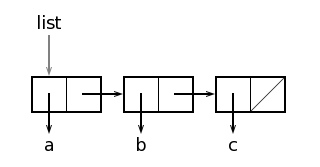The list consists of several of the cells linked with each other through pointers in the right part of cells. The right margin of the last cell of the list as a list terminating symbol refers to the empty list, i.e. on atom nil. Graphically the reference to the empty list often represent in the form of the crossed field. Pointers from first fields of cells of the list refer to the structures which are units of the list, in this case on atoms a, b and c.

## FIRST and REST select the pointer field

Operation of selectors first and rest in graphics representation becomes abundantly clear. If we apply function first to the list list contents of left margin of the first list cell will be result, i.e. a character:

```>(list  first)
a```

Accordingly call

```>(list  rest)
(b  c)```

returns value from right margin of the first list cell, i.e. the list (b c).

## CONS creates a cell and returns on it the pointer

Let's accept, that we have two lists:

```>(nil setq  head  '(b c))
nil
>(nil setq  tail  '(a b c))
nil```

Function call

```>(nil cons  head  tail)
((b c)  a b c)```

builds the new list of earlier constructed lists head and tail how it is shown in a figure.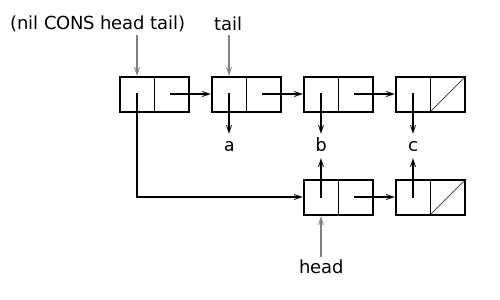cons creates a new list cell (and the list corresponding to it). Value of the first argument of call, and right - value of the second argument becomes contents of left margin of a new cell. Pay attention, that one new list cell can link two big structures in one new structure. This effective enough solution from the point of view of creation of structures and their representation in memory. We will notice, that function application cons has not changed structure of the lists which are arguments, and has not changed values of variables head and tail.

## Lists can have common parts

In one cell can specify one or more arrows from list cells, however one arrow can outgo from each field of a cell only. If on some cell there are some pointers this cell will describe the common subexpression. For example, in the list

(someone comes someone leaves)

symbol someone is the common subexpression to which pointers from first field from the first and from the third cell of the list refer.
If list units are not atoms, and sublists on a place of atoms will be there are first cells of sublists.
From a figure above it is visible, that logically identical atoms contain in system once, however logically identical lists can be presented various list cells. For example, values of calls

```>(nil setq  list  (nil  cons  head  tail))
nil
>(list  first)
(b  c)```

and

```>((list rest) rest)
(b  c)```

are logically identical list (b c) though they and are presented by various list cells:

```>((list first)  = ((list  rest) rest))
(b  c)```

However the list (b c) can consist and of the same cells.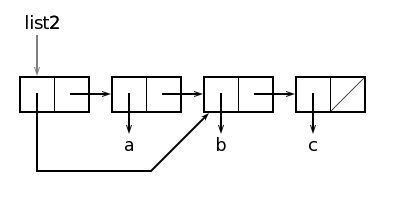This structure can be created by means of the following calls:

```>(nil setq  bc  '(b c))
nil
>(nil setq  abc (nil  cons  'a  bc))
nil
>(nil setq  list2 (nil  cons  bc  abc))
nil```

Thus, depending on a way of construction logical and physical structures of two lists can appear various. The logical structure always has the form of a binary tree while the physical structure can be the acyclic count, or, in other words, branches can converge again, but never can derivate the closed cycles, i.e. to specify back. Further we will see, that, using the pseudo-functions changing structures (field) ( setfirst, setrest and others), it is possible to create and cyclic structures.

## Logical and physical equality not same

Logically comparing lists, we used a predicate =, comparing not physical pointers, and coincidence of structural construction of lists and coincidence of the atoms forming the list.
Predicate eq it is possible to define physical equality of two expressions (i.e. whether values of arguments refer to one physical object) it is not dependent on, whether is it atom or the list. At matching of characters all the same, what predicate to use, as atomic objects are stored always in the same place. At matching by the list it is necessary to arrive more cautiously.
Function calls eq from a following example return as value false as logically identical arguments in this case are presented to memories by physically various cells:

```>(nil eq  '((b  c)  a b c)  '((b  c)  a b c))
false
>(nil eq  list  list2)
false
>(nil eq  (list first)  ((list  rest) rest))
false```

As parts first and rest the list list2 are presented by means of the same list cells we will receive the following result:

```>(nil eq  (list2  first)  ((list2 rest) rest))
(b  c)```

## The dotted pair corresponds to a list cell

Defining base function cons, we assumed, that its second argument is the list. This limitation is not necessary as by means of a list cell it would be possible, for example, result of call

(nil cons 'a 'b)

to present in the form of the structure represented in a figure.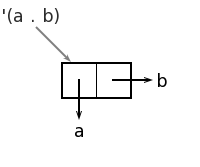In a figure is shown not the list, but more the common character expression, a so-called dotted pair. For matching in a figure we have represented the list (a b).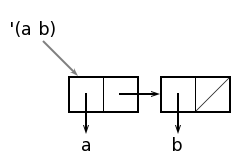The dotted pair name happens from the dot notation used in its record in which for sharing of first fields and rest the point selected with blanks is used:

```>(nil cons  'a  'b)
(a  . b)```

Expression to the left of a point (atom, the list or other dotted pair) corresponds to value of first field of a list cell, and expression to the right of a point - to value of rest field. Base functions first and rest operate absolutely symmetrically:

```>('(a . b)  first)  ; pay attention to the blanks selecting a point
a
>('(a . (b  . c)) rest)
(b  . c)```

The dot notation allows to expand a class of the objects represented by means of lists. The situation could be compared to the beginning of usage of fractions or complex numbers in arithmetics.

## Variants of dot and list records

Any list can be written in the dot notation. Conversion can be carried out (at all levels of the list) as follows:

(a1 a2 … an)

(a1 . (a2 . … (an . nil) … ))

Let's give an example:

(a b (c d) e)

(a . (b . ((c . (d . nil)) . (e . nil))))

For list tag here is nil in the field rest the last unit of the list, symbolising its termination.
The compiler can bring the expression written in the dot notation partially or completely in the list notation. Coercion is possible only when the dotted pair rest field is the list or pair. In this case it is possible to omit a point and brackets round the expression which are value of rest field:

 (a1 . (a2 a3)) ≡ (a1 a2 a3) (a1 . (a2 . a3)) ≡ (a1 a2 . a3) (a1 a2 . nil) ≡ (a1 a2 . ())≡ (a1 a2)

The point remains in expression only in case in the right part of pair there is an atom which is distinct from nil. To be convinced that the conversions made by the interpreter are true, it is possible, having drawn the structures corresponding to initial record and brought sort:

```>'(a  . (b  . (c  . (d))))
(a  b c d)
>'((a b)  . (b  c))
((a b)  b c)
>'(a  . nil)
(a)
>'(a  . (b  . c))
(a  b . c)
>'((((nil . a)  . b)  . c)  . d)
((((nil . a)  . b)  . c)  . d)```

Usage of dotted pairs in programming on a Lisp in general is excessive. With their help basically it is possible to reduce size of necessary memory some. For example data structure

(a b c)

written in the form of the list, demands three cells though the same data it is possible to present in sort

(a b . c)

demanding two cells. More compact representation can reduce a little and size of calculations for the score of smaller quantity of calls in memory.
Dotted pairs are applied in the theory, books and quick references on a Lisp. Often with their help designate the list of in advance unknown length in sort:

Dotted pairs are used together with some data types and with association lists, and also in system programming. The majority of programmers is not used by dotted pairs as in comparison with attentiveness demanded in that case the received scoring in a memory size and speed of calculations usually is not swept up.

## Memory management and garbage assemblage

As a result of calculations in memory there can be structures to which then it is impossible to refer. It happens when the calculated structure is not saved with the help setq or when the reference to old value as a result of a by-effect of new call setq or other function is lost. If, for example, to the list represented in a figure list3

```>(nil setq  list3
'((this will  garbage)  rest  part))
nil```

to assign new value

```>(nil setq  list3 (list3  rest))
nil```

that first-part as separates, as the pointer from atom list3 starts to refer how it is represented in a figure by means of a grey arrow.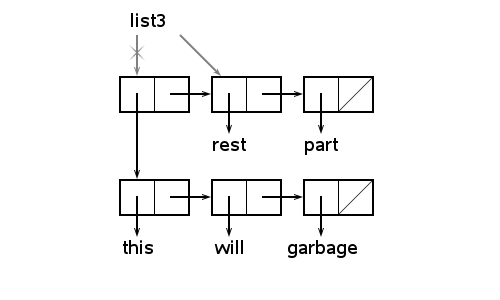Already it is impossible through symbols and pointers to reach four list cells. It is said that these cells became garbage.
Garbage arises and when the result of calculation does not contact any variable. For example, value of call

```>(nil cons  'a  (nil  list  'b))
(a  b)```

it is only printed, then the structure corresponding to it remains in memory garbage.
For a reuse of the memory which have become by garbage the special ashman who is automatically started when in memory there is not enough free space is provided. The ashman sorts out all cells and gathers cells being garbage in the list of free memory that they could be used anew.

## The calculations which are changing and not changing structure

All functions considered till now manipulated expressions, not calling any changes in already existing expressions. For example, function cons which like would change the arguments, actually builds the new list, functions first and rest in turn only select one of pointers. The structure of existing expressions could not vary as a function call by-effect. Values of variables could be changed only entirely, having calculated and having assigned new values entirely. The greatest, that thus could happen to old expression, is that it could disappear.
In a Lisp all the same there are also special functions with which help it is possible to influence structures of already existing expressions directly the same as, for example, in Pascal. It is carried out by functions, which as the surgeon, operate on internal structure of expressions.
Such functions name structure-destroying as with their help it is possible to break off structure and to paste together it in a new fashion.

## SETFIRST and SETREST change contents of fields

The main functions changing physical structure of lists, are setfirst and setrest which delete former and write new values in first fields and rest a list cell:

 (cell setfirst value-field) ;field first (cell setrest value-field) ;field rest

The object is the pointer on a list cell, argument - new value of first field written in it or rest. Both functions return as result the pointer on the changed list cell.

```>(nil setq  train '(locomotive1 a b c))
nil
>(train setfirst  'locomotive2)
(locomotive2  a b c)
>train
(locomotive2  a b c)
>((train  rest) setfirst  'tender)
(tender b c)
>train
(locomotive2  tender  b c)```

Function setrest is fulfilled the same as setfirst, with that difference that value of rest field varies:

```>(train setrest '(k l m))
(locomotive2  k l m)
>train
(locomotive2  k l m)```

Using function setrest, it is possible to define, for example, function circle, transforming any list in a ring:

```>(nil defmethod circle  ()  (this make-circle this))
(lambda nil (this make-circle this))
>(nil defmethod make-circle (x)
(nil  cond
((nil atom  this) this)
((nil atom  (this rest))  (this setrest x))
(true ((this  rest) make-circle x))))
(lambda (x) (nil  cond  ((nil atom  this) this) ((nil atom  (this rest))  (this setrest x)) (true ((this  rest) make-circle x))))
>('(a b c)  circle)
(c  a b … c a b … )```

## Modify structure can accelerate calculations

Functions reasonably used structure-destroying can, as well as the knife of the surgeon to be effective and useful tools. Further we for an example will consider, how it is possible to raise with the help structure-destroying of pseudo-functions efficiency of function + for lists. This function unites the lists which are its arguments in one list:

```>(nil setq  begin '(a b))
nil
>(nil setq  end '(c d))
nil
>(nil setq  result  (begin  + end))
nil```

Can seem, that the call brought above +, as though changes pointers how it is shown by grey arrows.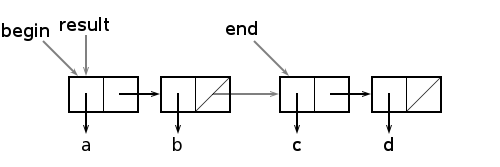However it is not true, as value of the list begin cannot vary, as + the structure-destroying function is not. Only new value result is calculated and assigned. We will receive the structures represented in a figure: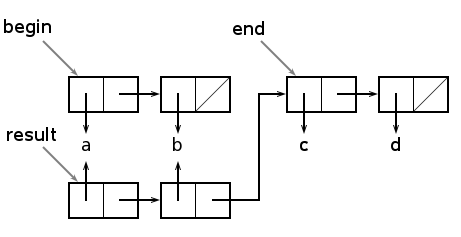From a figure it is visible, that + creates a copy of the list which is the first argument. If this list very long calculations will be long also. Creation of list cells by means of function cons demands time and in the future adds operations to the ashman. If, for example, the list begin contains 1000 units, and end - one unit during calculation 1000 new cells though the question consists only in addition of one unit to the list will be created. If the arrangement of arguments was another there would be one cell, and lists would be united approximately in 1000 times faster.
If it is not essential to us, that the value begin will vary, we can instead of function + use faster function +=. Function += makes the same, as +, with that only a difference, that it simply unites lists, changing the pointer in the field rest the last cell of the list which is the first argument, on the beginning of the list which is the second argument as it is shown in the first figure:

```>('begin  set '(a b))
(a  b)
>('end  set '(c d))
(c  d)
>('result set (begin  +=  end)) ; destroying association
(a  b c d)
>begin  ; by-effect
(a  b c d)
>end
(c  d)```

Having used function +=, we have avoided list copying begin, however as a by-effect the value begin has varied. As all other structures, using cells of an initial value begin will vary also.
The pseudo-functions similar setfirst, setrest and +=, changing internal structure of lists, are used in the event that it is necessary to make little changes in the big data structure. With the help structure-destroying of functions it is possible to change effectively for once at once some structures if these structures have sublattices combined in memory.
Artful by-effects, like change of values of external variables, can lead to surprises in those parts of the program which do not know about changes. It complicates a spelling, documenting and support of programs. Generally the functions changing structures, try not to use.